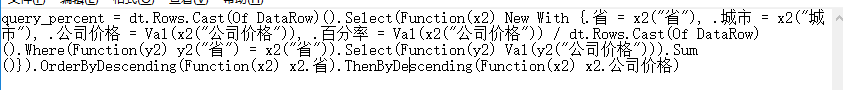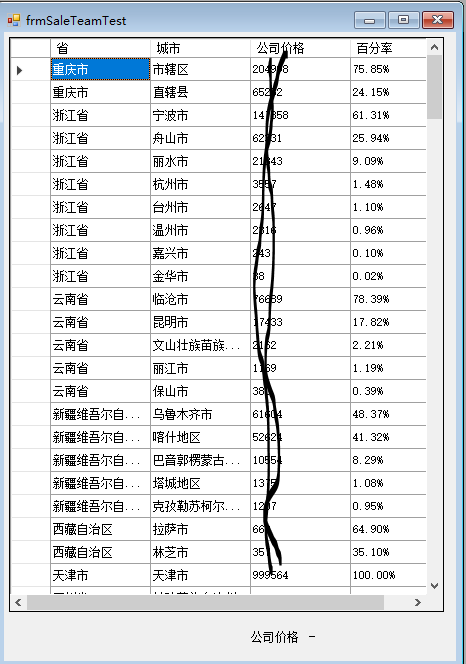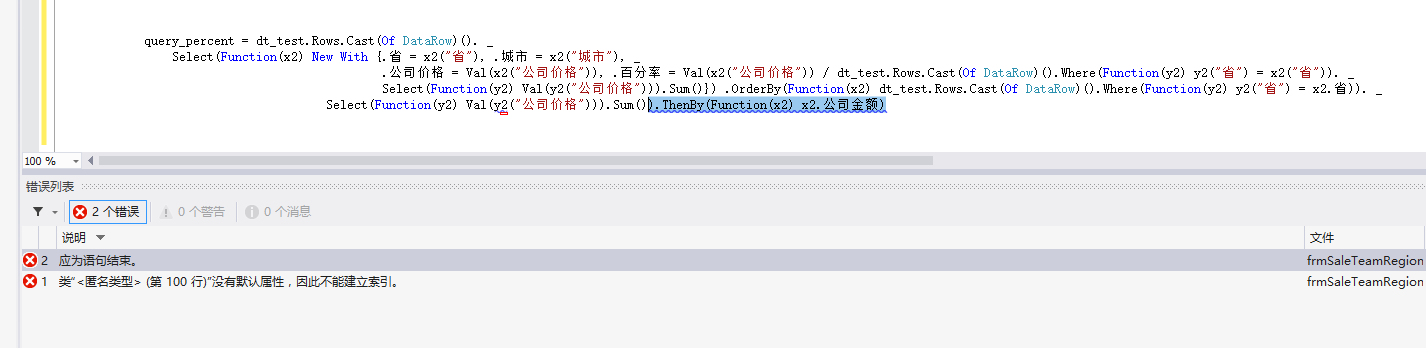vb.net dataTable使用group by 及排序问题 5C2个回答

.OrderBy(Function(x) x.省别).ThenBy(Function(x) x.金额)

.OrderBy(Function(x) dataTable.Rows.Cast(Of DataRow)().Where(Function(y) y("省别") = x.省别)).Select(Function(y) Val(y("金额"))).Sum() ).ThenBy(Function(x) x.金额)weixin_40187983 这个问题我还是没有搞定weixin_40187983 query_percent = dt_test.Rows.Cast(Of DataRow)().Select(Function(x2) New With {.省 = x2("省"), .城市 = x2("城市"), .公司价格 = Val(x2("公司价格")), .百分率 = Val(x2("公司价格")) / dt_test.Rows.Cast(Of DataRow)().Where(Function(y2) y2("省") = x2("省")).Select(Function(y2) Val(y2("公司价格"))).Sum()}) .OrderBy(Function(x2) dt_test.Rows.Cast(Of DataRow)().Where(Function(y2) y2("省") = x2.省)).Select(Function(y2) Val(y2("公司价格"))).Sum()).ThenBy(Function(x2) x2.公司金额)weixin_40187983 还是有报错，请你看一下那边的问题